# Texas Go Math Grade 1 Lesson 2.2 Answer Key Less Than

Refer to our Texas Go Math Grade 1 Answer Key Pdf to score good marks in the exams. Test yourself by practicing the problems from Texas Go Math Grade 1 Lesson 2.2 Answer Key Less Than.

## Texas Go Math Grade 1 Lesson 2.2 Answer Key Less Than

Essential Question
How can you compare two numbers to find which is less?
Explanation:
First we will check the tens then ones
When the digits in hundreds place are equal, then the number with more number of tens is a greater number. 4. When the digits in tens place are equal, then the number with more number of ones is a greater number.

Explore.
Useto solve. Draw quick pictures to show your work.For The Teacher

• Read the problem. Which number is less, 22 or 28? Have children use base-ten blocks to solve.
Explanation:
Compared the tens as tens are same
compared the ones
2  < 8Model and Draw
Compare numbers to find which is less.Explanation:
Compared the tens as tens are same
compared the ones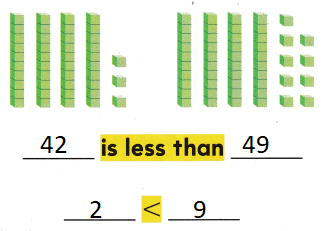Share and Show

Useto show each number.

Question 1.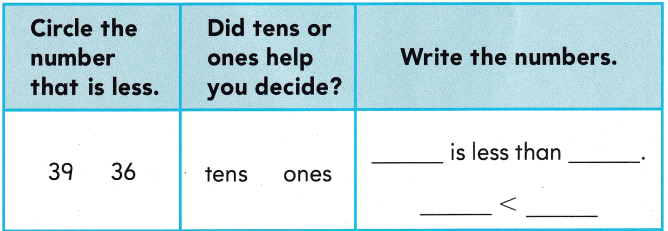Explanation: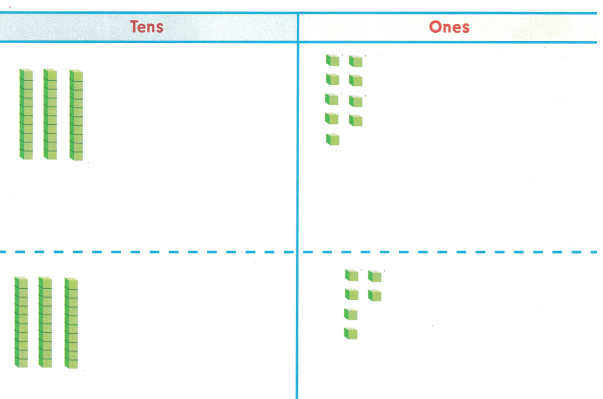Compare the tens as they are equally
compared the ones

Question 2.Explanation:Compare the tens as they are equally
compared the ones

Question 3.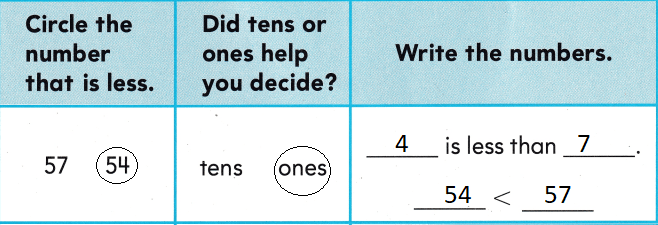Explanation: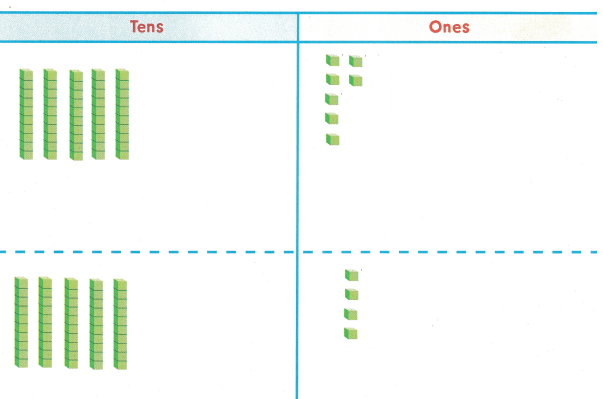Compare the tens as they are equally
compared the ones

Problem Solving

Question 4.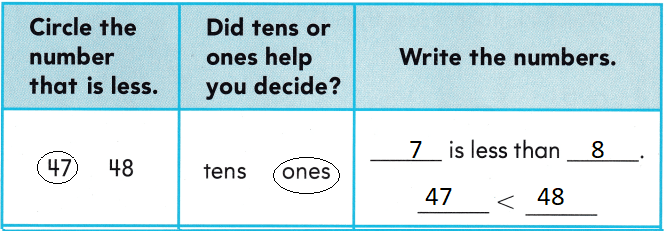Explanation:Compare the tens as they are equally
compared the ones

Question 5.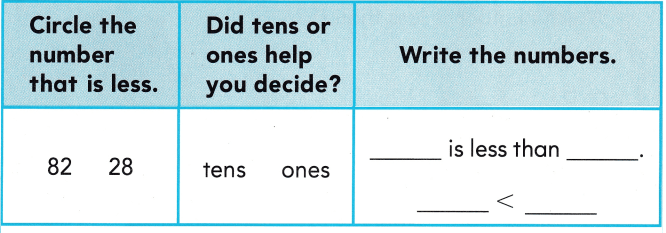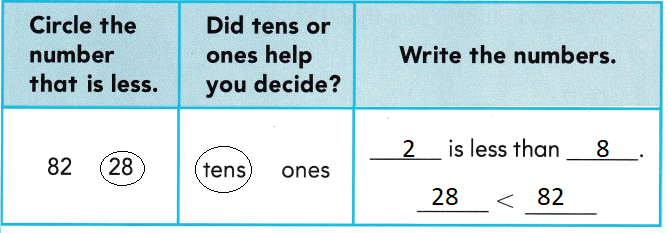Explanation:Compare the tens as they are equally
compared the ones

Write a number to solve.

Question 6.
Jack makes the number 2. Kit makes a number that has fewer ones than 92 and fewer tens than 5 tens. What could be a number Kit makes?Explanation:
Compare the tens
Jack makes the number 2.
Kit makes a number that has fewer ones than 92 and fewer tens than 5 tens.
The number might be 41
1 < 2
4 < 5

Question 7.
H.O.T. Multi-Step Ella has 22 beads. Lauren has 48 beads. Aya has fewer than either Ella or Lauren. How many beads might Aya have?
Explanation:
Compare the tens
Aya has fewer than either Ella or Lauren.
That might be 21
21 < 22

Question 8.
A spider catches 15 flies today. Which number is less than 15?(A) 42
(B) 51
(C) 11
Explanation:
Compare the tens as the tens are same
compared the ones
11 < 15

Question 9.
Analyze Which number is less than 42?
(A) 22
(B) 50
(C) 79
Explanation:
Compare the tens
22 < 42

Question 10.
Which number is less than 63?
(A) 102
(B) 36
(C) 93
Explanation:
Compare the tens
36 < 63

Question 11.
Texas Test Prep Which number is less than 72?
(A) 49
(B) 99
(C) 80
Explanation:
Compare the tens
49 < 72

Take Home Activity

• Write 47, 54, 57, and 74 on slips of paper. Show your child two numbers, and ask which number is less. Repeat with different pairs of numbers.
Explanation:
47 and 54
47 < 54
54 and 57
54 < 57
57 and 74
57 < 74

### Texas Go Math Grade 1 Lesson 2.2 Homework and Practice Answer Key

UseQuestion 1.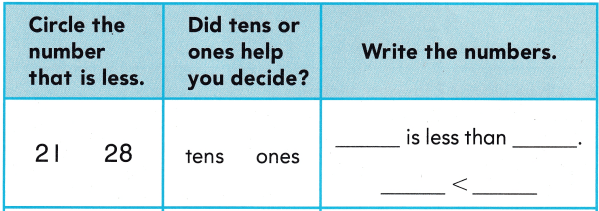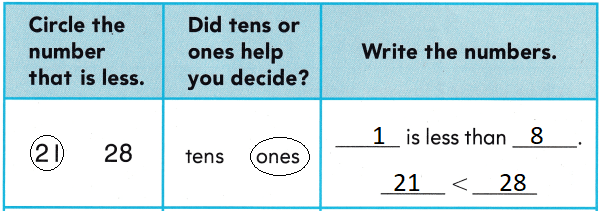Explanation: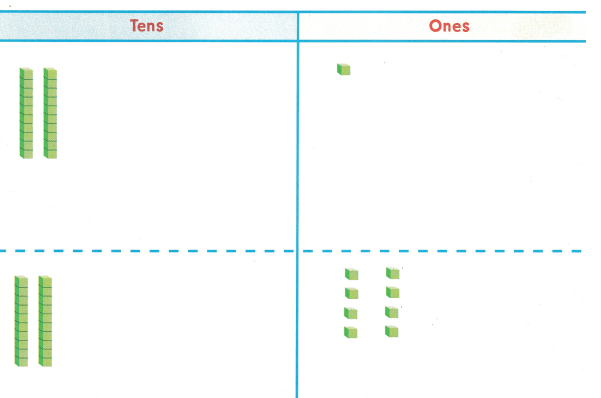Compare the tens as they are equally
compared the ones

Question 2.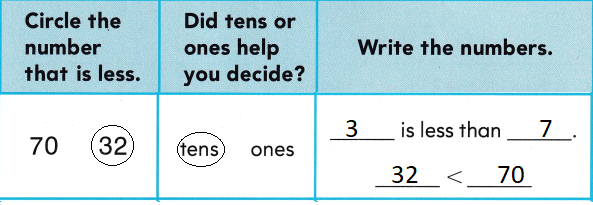Explanation:Compare the tens as they are equally
compared the ones

Question 3.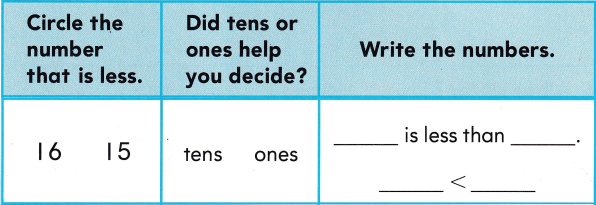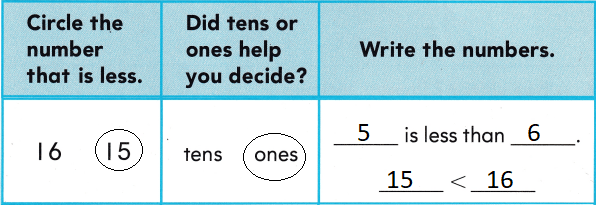Explanation:Compare the tens as they are equally
compared the ones

Problem Solving

Write or draw to solve.

Question 4.
Jed has 25 toy cars. Kai has 32 toy cars. Ken has fewer cars than either Jed or Kai. How many cars might Ken have?__ cars
24 < 25
Explanation:
Jed has 25 toy cars. Kai has 32 toy cars.
Ken has fewer cars than either Jed or Kai.
The number might be 24
24 cars might Ken have

Texas Test Prep

Lesson Check

Question 5.
A squirrel hides 21 nuts. Which number is less than 21?
(A) 20
(B) 25
(C) 30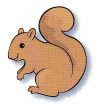Explanation:
Compare the tens as they are equally
compared the ones
20 < 21

Question 6.
A bee visits 67 flowers today. Which of these numbers is less than 67?
(A) 76
(B) 68
(C) 58
Explanation:
Compare the tens
58 < 67

Question 7.
Which of these numbers is less than 15?
(A) 25
(B) 4
(C) 19
Explanation:
Compare the tens
15 < 4

Question 8.
Which number is less than 81?
(A) 71
(B) 91
(C) 88